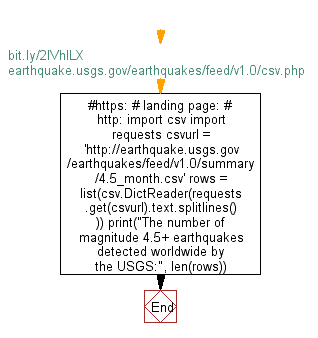# Python Web Scraping: Get the number of magnitude 4.5+ earthquakes detected worldwide by the USGS

## Python Web Scraping: Exercise-25 with Solution

Write a Python program to get the number of magnitude 4.5+ earthquakes detected worldwide by the USGS.

Sample Solution:-

Python Code:

``````#https://bit.ly/2lVhlLX
# landing page:
# http://earthquake.usgs.gov/earthquakes/feed/v1.0/csv.php
import csv
import requests
csvurl = 'http://earthquake.usgs.gov/earthquakes/feed/v1.0/summary/4.5_month.csv'
print("The number of magnitude 4.5+ earthquakes detected worldwide by the USGS:", len(rows))
```
```

Output

```The number of magnitude 4.5+ earthquakes detected worldwide by the USGS: 397
```

Flowchart:Python Code Editor:

Have another way to solve this solution? Contribute your code (and comments) through Disqus.

What is the difficulty level of this exercise?

﻿

## Python: Tips of the Day

Python: The Zip() Function

```>>> students = ('John', 'Mary', 'Mike')
>>> ages = (15, 17, 16)
>>> scores = (90, 88, 82, 17, 14)
>>> for student, age, score in zip(students, ages, scores):
...     print(f'{student}, age: {age}, score: {score}')
...
John, age: 15, score: 90
Mary, age: 17, score: 88
Mike, age: 16, score: 82
>>> zipped = zip(students, ages, scores)
>>> a, b, c = zip(*zipped)
>>> print(b)
(15, 17, 16)
```